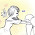### Wondrous Tails Solver

Click to add / remove seals (0 / 9)

Strategy:

At leastChance
1 line
2 lines
3 lines

### Strategy Performance and Comparison

Second chance points:
0%

### How to use

#### TLDR

1. Click on the journal and add 7 seals.
2. The "shuffle: yes / no" verdicts will appear under "Shuffle Recommendation".
• Follow the one that corresponds to the number of second chance points that you have or are planning to spend before you turn in your journal.
3. The default strategy (1 line maximum chance) is a good choice.
• To see how it compares to other strategies, click on "Simulate" to calculate the expected chance of getting 1, 2 and 3 lines when following the recommendations of each strategy.

#### Choosing a strategy

Each strategy optimizes for a different objective.
• 1 line maximum chance: geared towards maximizing the chances of getting 1 line, disregarding what happens to the chances of getting 2 and 3 lines.
• 2 lines maximum chance: geared towards maximizing the chances of getting 2 lines, disregarding what happens to the chances of getting 1 and 3 lines.
• 1 and 2 lines tradeoff: geared towards balancing the chances of getting 1 and 2 lines. Journals with 2 lines are valued 4 times as much as journals with 1 line.
• 3 lines chance: geared towards maximizing the chances of getting 3 lines, disregarding what happens to the chances of getting 1 and 2 lines.
• Potential Lines: the strategy described here.
• Never Shuffle: always recommends not to shuffle.
The three meaningful choices here are "1 line maximum chance", "2 lines maximum chance" and "1 and 2 lines tradeoff". At 8-9 seals, switching between those 3 strategies involves trading up to ~6% of your chances of getting at least 1 line with up to ~1% of your chances of getting at least 2 lines. Trade-off's worth depends on the nature of the rewards and how much you value them. Run a simulation from an empty journal for details.
Getting 3 lines is a rare event. If you're feeling lucky or you like gambling feel free to use "Maximize 3 lines chance".
"Potential Lines" is a popular strategy that is easy to mentally track. The purpose of including it in the list is to reassure those who followed it at some point in the past that they're getting better results with the other strategies.
"Never Shuffle" is useful as a benchmark for the other strategies and its numbers should be similar to the ones in the "Chances without shuffling" section.

### How it works

#### Simulation

"Simulate" uses the specified second chance points to run 100,000 simulations for each strategy. Each simulation run starts from the specified journal. If fewer than 7 seals are present, random ones will be added in each run till 7 is reached. At 7 seals, the recommendation of the strategy being simulated is applied till we run out of second chance point (or the strategy recommends "no shuffle"). The results of the simulation describe the percentage of simulation runs where each strategy produced a journal with at least 1, 2 and 3 lines. If the number of seals in the specific journal is 7, an additional column that shows its recommendations will be added. You can run the simulation from an empty board to see how well each strategy performs in general.

#### Maximum lines strategies

To understand how those strategies work, let's start with a simpler version. We're given a six-sided die and we're allowed to roll it up to $r$ times. Our goal is to get the highest die roll that we possibly can. We can stop rolling at any point, but we can't go back to a previous roll. We're asked to come up with a strategy (reroll / don't reroll) that would give us the highest expected value for the die. The maximum expected value can be calculated as follows:
$f(x, r) = \begin{cases} x, & \text{if r = 0} \\ max \begin{cases} \frac{\displaystyle\sum_{i=1}^n f(face_i, r - 1)}{n} \\ x \end{cases}, & \text{if r \gt 0} \end{cases}$ Where $x$ is the current roll (1..6), $r$ is the remaining number of rerolls, $n$ is the number of faces (6) and $face_i$ is the value on the $i^{th}$ face (1, 2, 3, 4, 5, 6).
The "reroll / don't reroll" decision for a given state depends on which value of the arguments to $max$ is higher. If $x$ has the higher the value, then the better decision is "don't reroll". If the other argument has the higher value, then the better decision is "reroll".
The Wondrous Tails shuffling problem can be reduced to the die rolling problem, except in this case we have 11440 faces (the number of different journals with 7 seals) and each face can have a value between 0 and 72 (the number of ways we can place the last 2 seals to satisfy our objective). The 4 objectives we use here are "at least 1 line", "at least 2 lines", "1 and 2 lines tradeoff" and "3 lines". For the "1 and 2 lines tradeoff" objective / strategy, the value on each face / 7-seals-journal is $a + 4 * b$, where $a$ is the number of ways to get 1 line with the last 2 seals and $b$ is the number of ways to get 2 lines with the last 2 seals.

### Credits

Lyaa: The developer of this calculator who was kind enough to let us reuse its graphics.

### Comments

1.Request: can there be an option show the shuffle suggestions for ALL strategies in a single table?

1.Thanks! That's a good suggestion. I'm worried that it might be more confusing to show multiple (potentially conflicting) recommendations for users who are looking for a quick answer.

Having that said, I like the idea of making it optional (perhaps a "show all" link / button). I'll see if I can do something there.